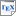[KT14] Marta Kwiatkowska and Chris Thachuk. Probabilistic Model Checking for Biology. In Software Safety and Security, IOS Press. 2014. [pdf] [bib] [A tutorial on the application of probabilistic model checking and PRISM to biological systems, including examples of DNA computation.] Downloads:pdf (980 KB)bib Links: [Google] [Google Scholar] [CiteSeer] Abstract. Probabilistic model checking is an automated method for verifying the correctness and performance of probabilistic models. Property specifications are expressed in probabilistic temporal logic, denoting, for example, the probability of a given event, the probability of its occurrence within a given time interval, or expected number of times it has occurred in a time period. This chapter focuses on the application of probabilistic model checking to biological systems modelled as continuous-time Markov chains, illustrating the usefulness of these techniques through relevant case studies performed with the probabilistic model checker PRISM. We begin with an introduction to discrete-time Markov chains and the corresponding model checking algorithms. Then continuous-time Markov chain models are defined, together with the logic CSL (Continuous Stochastic Logic), and an overview of model checking for CSL is given, which proceeds mainly by reduction to discrete-time Markov chains. The techniques are illustrated with examples of biochemical reaction networks, which are verified against quantitative temporal properties. Next a biological case study analysing the Fibroblast Growth Factor (FGF) molecular signalling pathway is summarised, highlighting how probabilistic model checking can assist in scientific discovery. Finally, we consider DNA computation, and specifically the DSD formalism (DNA Strand Displacement), and show how errors can be detected in DNA gate designs, analogous to model checking for digital circuits.